# Electronics and Communication Engineering - Exam Questions Papers

36.

In the following transistor circuit VBE = 0. 7 V, rc = 25mV/IE, and β and the capacitance are very large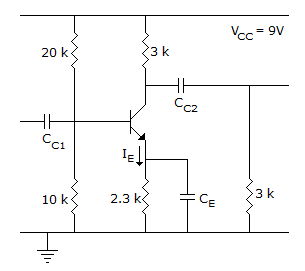The value of DC current IE is

 A. 1 mA B. 2 mA C. 5 mA D. 10 mA

Explanation:

Design is independent of IB as β is very high

IB ∼ 0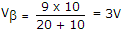Thus applying Kirchhoff's law is base emitter, we get.

37.

A certain JK FF has tpd = 12 nsec. The largest MOD counter that can be constructed from these FFs and still operate up to 10 MHz is

 A. any B. 8 C. 256 D. 10

Explanation: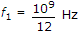f0 = 107 Hz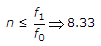⇒ 8.33

n = 8.

38.

A second-order system has a transfer function is given by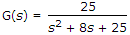. If the system, initially at rest is subjected to a unit step input at t = 0, the second peak in response will occur at :

 A. p sec B. p/3 sec C. p/6 sec D. p/2 sec

Explanation: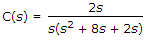Peak time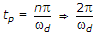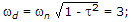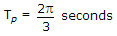.

39.

Value of ZL for maximum power transfer is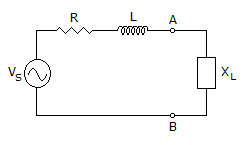A. R B. R + jωL C. R - jωL D. None of these

Explanation:

The value of load for maximum power transfer is given by complex conjugate of ZAB. ZAB = R + jXL = R + jωL.

ZL for maximum power transfer is given by, ZL = R - jωL.

40.

A periodic voltage has following values for equal time intervals changing suddenly from one value to next : 0, 5, 10, 20, 50, 60, 50, 20, 10, 5, 0, - 5, - 10 etc.
RMS value of the waveform is :

 A. 31 v B. 32 v C. insufficient data D. none of these

Explanation:

Mean value of V2 =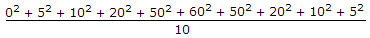= 965 V

rms value =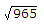≈ 31 V.

#### Current Affairs 2022

Interview Questions and Answers